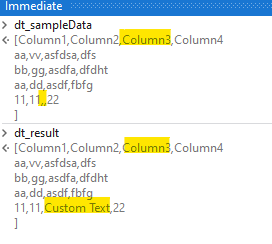# If Empty Cell Write Text Based on Column Name

Hi…

i did any mistake on below code…

(From row In dt.AsEnumerable()
Let loa = row.ItemArray.ToList().row.Field(Of String)(“Product”)
Let ra = loa.ConvertAll(Function(a) If(IsNothing(a) OrElse String.IsNullOrEmpty(a.ToString),“Null Data”,a))

Try to write empty cell in the datatable write “Null Data” Based on the Column Name…

Thanks
Shyam

There is a mistake here:

row.ItemArray.ToList().row.Field(Of String)(“Product”)

Kind Regards,
Ashwin A.K

Ok…

i missed any commands ?

can you check and update the code ?

Thanks
Shyam

Since you are only interested in updating a single column, then this might be what you are looking for:Assign → str_colName

``````str_colName = "Column3"
``````
``````(From row In Dt_sampleData.AsEnumerable()
Let colInd = Dt_sampleData.Columns(str_colName).Ordinal
Let col = If(IsNothing(row(str_colName)) OrElse String.IsNullOrEmpty(row(str_colName).ToString),"Custom Text", row(str_colName))
Let ra = row.ItemArray.Take(colInd).Append(col).Concat(row.ItemArray.Skip(colInd+1)).ToArray()
``````

UpdateOnlyColumn.xaml (8.0 KB)

Kind Regards,
Ashwin A.K

1 Like

Thanks…

if Multiple Columns means where i change the code…

Thanks
Shyam

We are keeping track of the Column Index using the Ordinal Method as we manipulate the data, so that after we are done we can place the edited column values in the exact same column index.

Trying to make adjustments so that it will accommodate multiple columns will just cause a mess, so I’d recommend reusing the same code for other columns as well.

If you wish to apply the condition for all columns, then you can use the ConvertAll Method from earlier

``````(From row In dt.AsEnumerable()
Let loa = row.ItemArray.ToList()
Let ra = loa.ConvertAll(Function(a) If(IsNothing(a) OrElse String.IsNullOrEmpty(a.ToString),“Null Data”,a))

``````

I hope that answers your question, and also could you please verify if the code works as expected?

Kind Regards,
Ashwin A.K

it working fine after changing the code.

(From row In dt.AsEnumerable()
Let loa = row.ItemArray.ToList()
Let ra = loa.ConvertAll(Function(a) If(IsNothing(a) OrElse String.IsNullOrEmpty(a.ToString),“Null Data”,a)).ToArrya()